Question

I know that the answer is a but what are the steps of solving it?? Q11...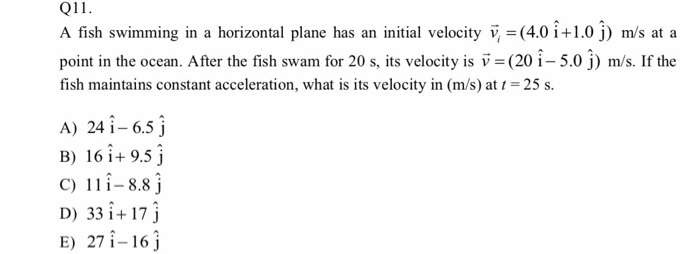I know that the answer is a but what are the steps of solving it??
Q11 A fish swimming in a horizontal plane has an initial velocity v, (4.0 i+1.0 j) m/s at a point in the ocean. After the fish swam for 20 s, its velocity is v (20î- 5.0 j) m/s. If the fish maintains constant acceleration, what is its velocity in (m/s) at-25 s A) 24 i 6.5 j B) 16i.+ 9.5 j C) 11 i-8.8 j D) 331+17 j E) 271-16j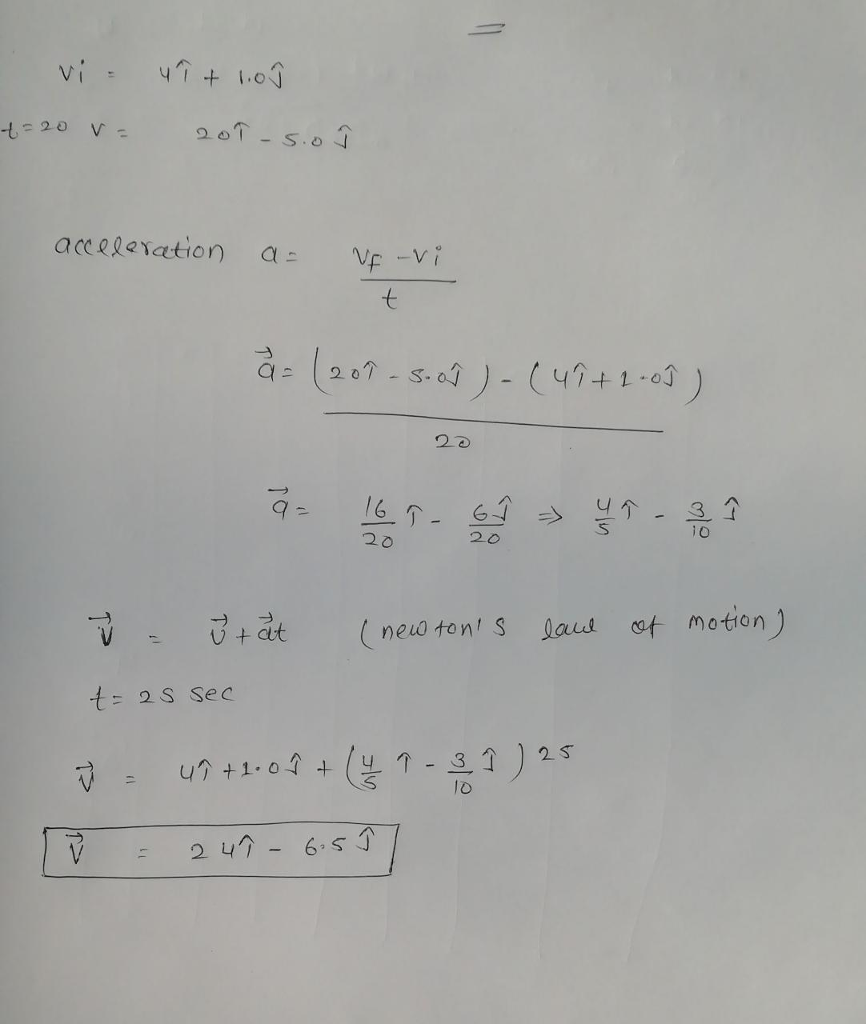Earn Coins

Coins can be redeemed for fabulous gifts.

Similar Homework Help Questions
• Please help! A fish swimming in a horizontal plane has velocity v = (4.00 i +...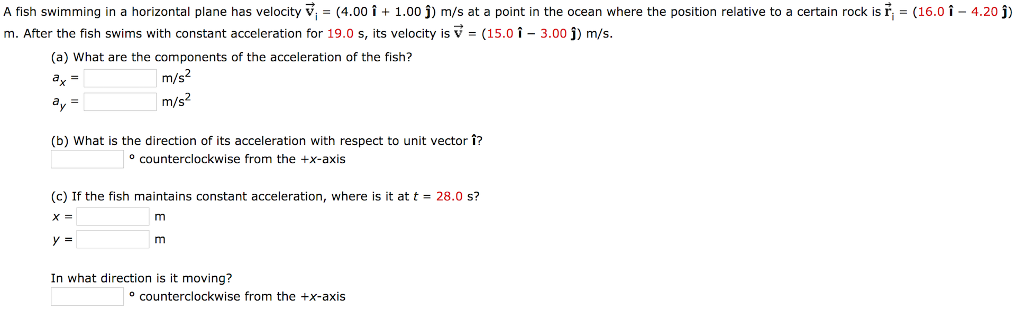Please help! A fish swimming in a horizontal plane has velocity v = (4.00 i + 1.00 j) m/s at a point in the ocean where the position relative to a certain rock is ri = (16.0-4.20 j) m. After the fish swims with constant acceleration for 19.0 s, its velocity is V (15.0 1-3.00 j) m/s. (a) What are the components of the acceleration of the fish? m/s m/s (b) What is the direction of its acceleration with respect...

• A fish swimming in a horizontal plane has velocity v with arrowi = (4.00 i +...

A fish swimming in a horizontal plane has velocity v with arrowi = (4.00 i + 1.00 j) m/s at a point in the ocean where the position relative to a certain rock is r with arrowi = (14.0 i − 4.20 j) m. After the fish swims with constant acceleration for 19.0 s, its velocity is v with arrow = (15.0 i − 3.00 j) m/s. (a) What are the components of the acceleration of the fish? ax =...

• A fish swimming In a horlzontal plane has velocity v, (4.00 i5.00 j) m/s at a...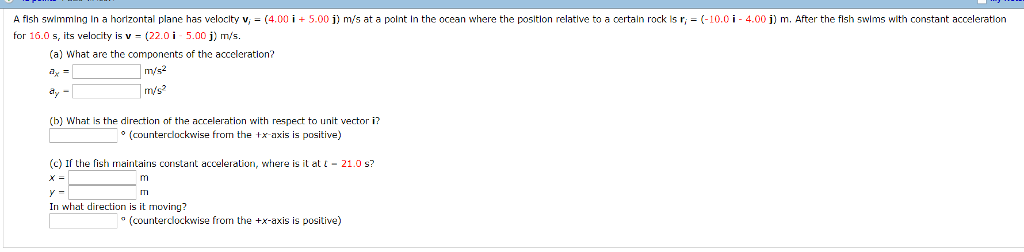A fish swimming In a horlzontal plane has velocity v, (4.00 i5.00 j) m/s at a polnt In the ocean where the positlon relatlve to a certain rock Is r(10.0 i 4.00 ) m. After the fish swlms wth contant acceleratlon for 16.0 s, its velocity is v (22.0i 5.00 j) m/s. (a) What are the components of the acceleration? ritt? (b) What is the direction of the acceleration with respect to unit vectori? (counterdlockwise from the x axis is...

• I know that the answer is a but what are the steps of solving it?? 915....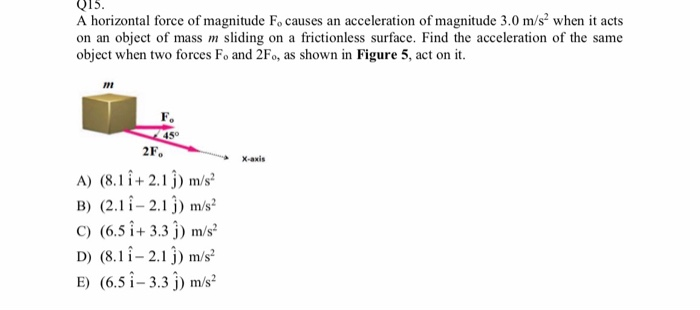I know that the answer is a but what are the steps of solving it?? 915. A horizontal force of magnitude Fo causes an acceleration of magnitude 3.0 m/s2 when it acts on an object of mass sliding on a frictionless surface. Find the acceleration of the same object when two forces Fo and 2Fo, as shown in Figure 5, act on it. n in Pigure 5, ari on ih Fo 450 2Fo X-axis A) (8.1 i+ 2.1j) m/s B)...

• A fish swimming in a horizontal plane has velocity v.- 4.00 + 1.00 j m s...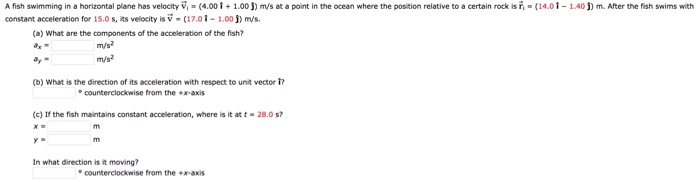A fish swimming in a horizontal plane has velocity v.- 4.00 + 1.00 j m s at a point in the ocean where the post on relative to a certain rock sn· constant acceleration for 15.0 s, its velocity is v (01 1.00j) m/s. 14,0 1-140 m. After the sh swims wit (a) What are the components of the acceleration of the fish? ay (b) What is the direction of its acceleration with respect to unit vector ? ecounterclockwise from...

• If the number is a decimal please include 4 digits past the decimal point if available....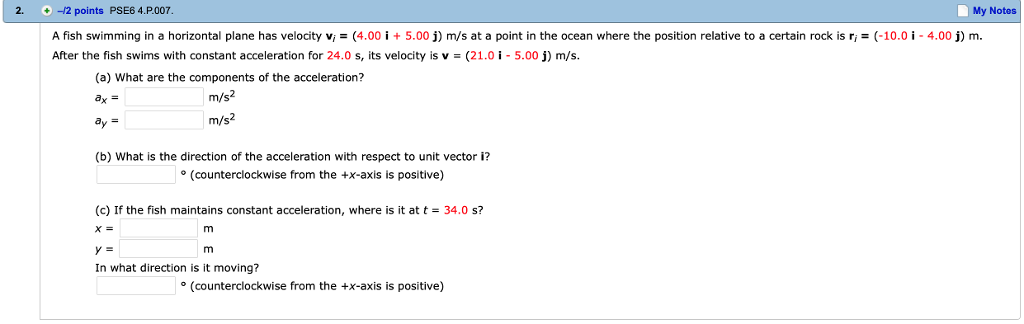If the number is a decimal please include 4 digits past the decimal point if available. 2. -12 points PSE6 4.P.007 My Notes A fish swimming in a horizontal plane has velocity vi = (4.00 İ + 5.00 j) m/s at a point in the ocean where the position relative to a certain rock is ri = (-10.01-4.00 j) m. After the fish swims with constant acceleration for 24.0 s, its velocity is v-(21.0-5.00) m/s. (a) What are the components...

• just the last one idk why its wrong A fish swimming in a horizontal plane has...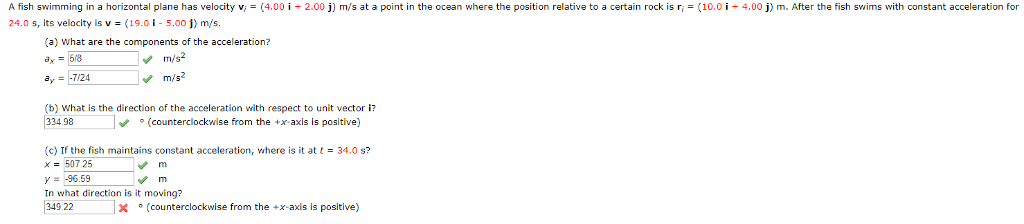just the last one idk why its wrong A fish swimming in a horizontal plane has velocity v 24.0 s, its velocity is v(19.01-5.001) m/s 4.00 і 2.00 j) m s at a point in the ocean where the position relat ve to a certan rocks 4.00 m After the ist swi snitt constan acceleration (a) What are the components of the acceleration? a,-58 m/s2 (b) what is the direction of the acceleration with respect to unit vector i 334...

• Help! A fish swimming in a horizontal plane has velocity V (4.00 i1.00 j) m/s at...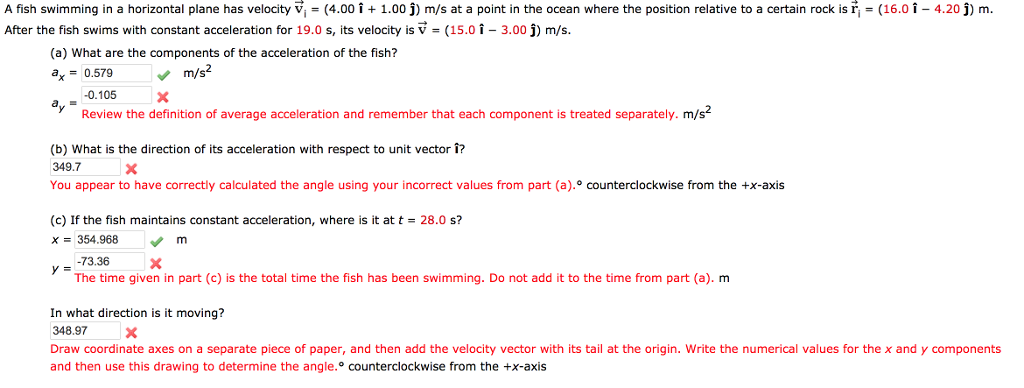Help! A fish swimming in a horizontal plane has velocity V (4.00 i1.00 j) m/s at a point in the ocean where the position relative to a certain rock is fter the fish swims with constant acceleration for 19.0 s, its velocity is V (15.0 1-3.00 j) m/s (16.0 1 4.20) m (a) What are the components of the acceleration of the fish? ax0.579 m/s2 0.105X Review the definition of average acceleration and remember that each component is treated separately....

• 2O6 points SerPSE9 4.P.009 MLFB My Notes Ask Your h swimming in a horizontal plane has...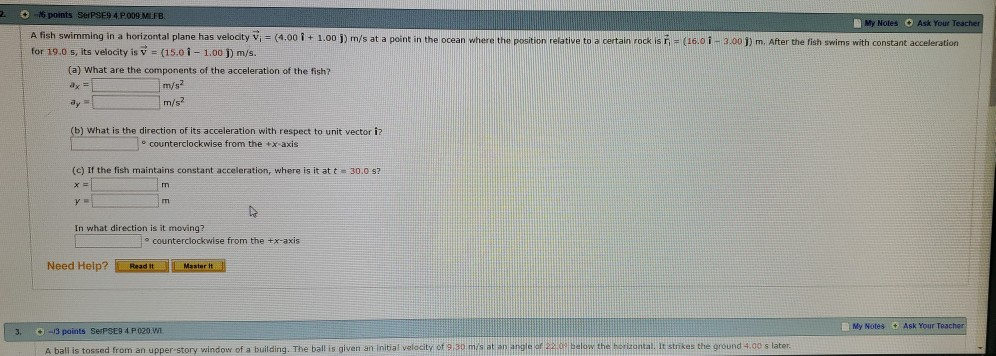2O6 points SerPSE9 4.P.009 MLFB My Notes Ask Your h swimming in a horizontal plane has velocity Vi (4.00 i + 1.00 j) m/s at a point in the ocean where the position relative to a certain rock isi- (16.01 -3.00 1) m. After the fish swims with constant acceleration for 19.0 s, its velocity is v (15.01-1.00 j) m/s. (a) What are the components of the acceleration of the fish? m/s2 m/s2 b) What is the direction of its...

• Please answer all. The displacement vectors A and B shown in the figure below both have...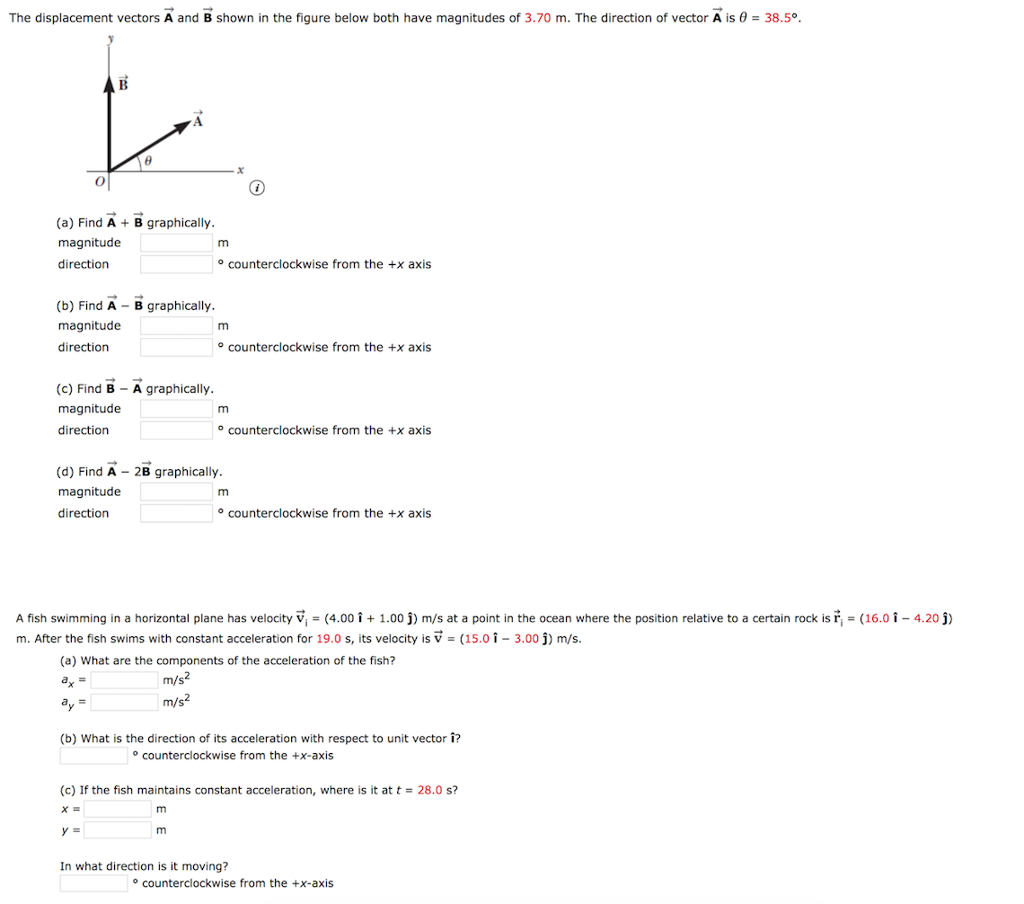Please answer all. The displacement vectors A and B shown in the figure below both have magnitudes of 3.70 m. The direction of vector A is θ = 38.5° (a) Find A B graphically magnitude direction o counterclockwise from the +x axis (b) Find A B graphically magnitude direction o counterclockwise from the +x axis (c) Find B A graphically magnitude direction o counterclockwise from the +x axis (d) Find A 2B graphically magnitude direction o counterclockwise from the +x...